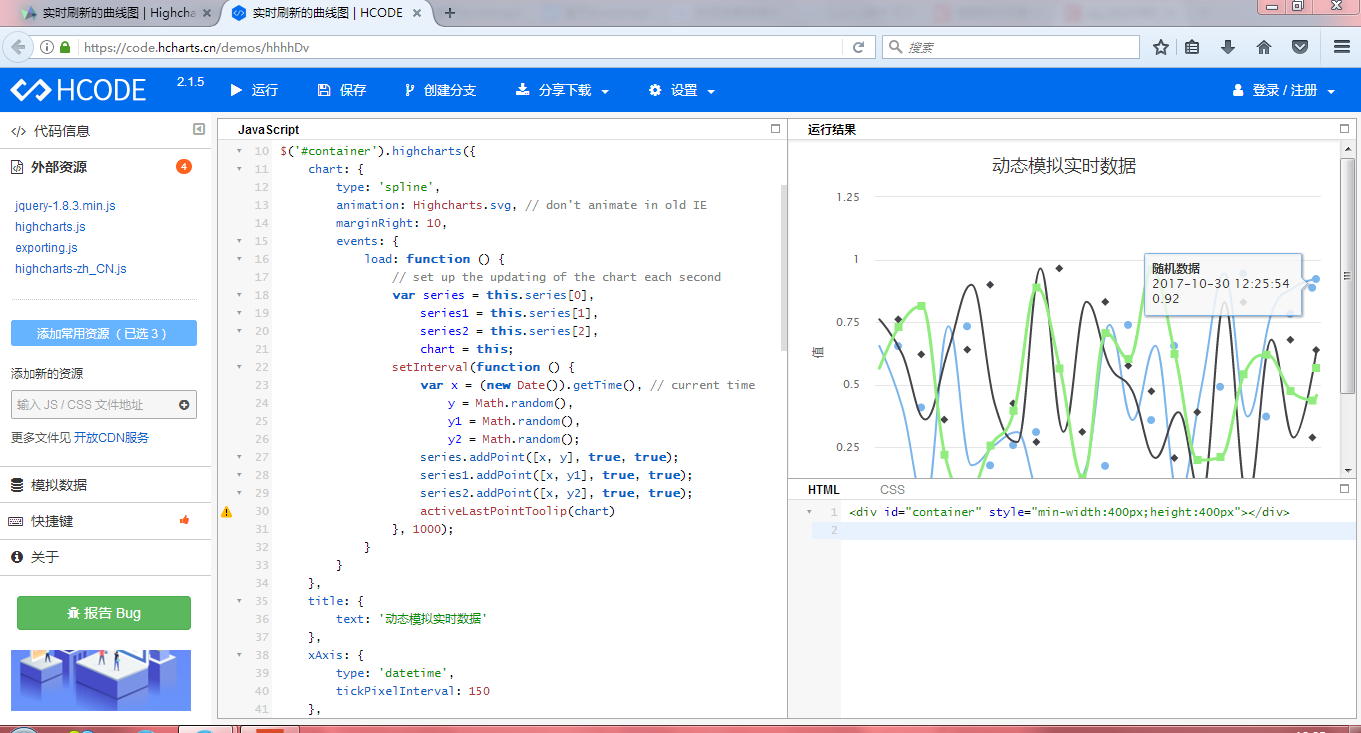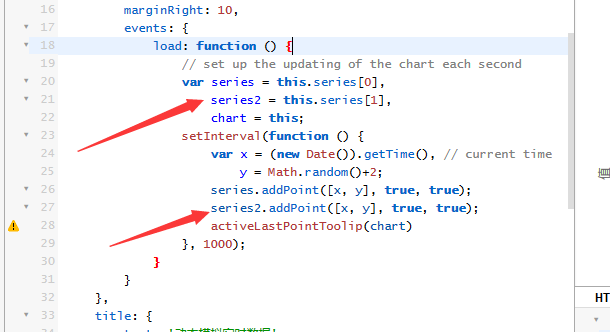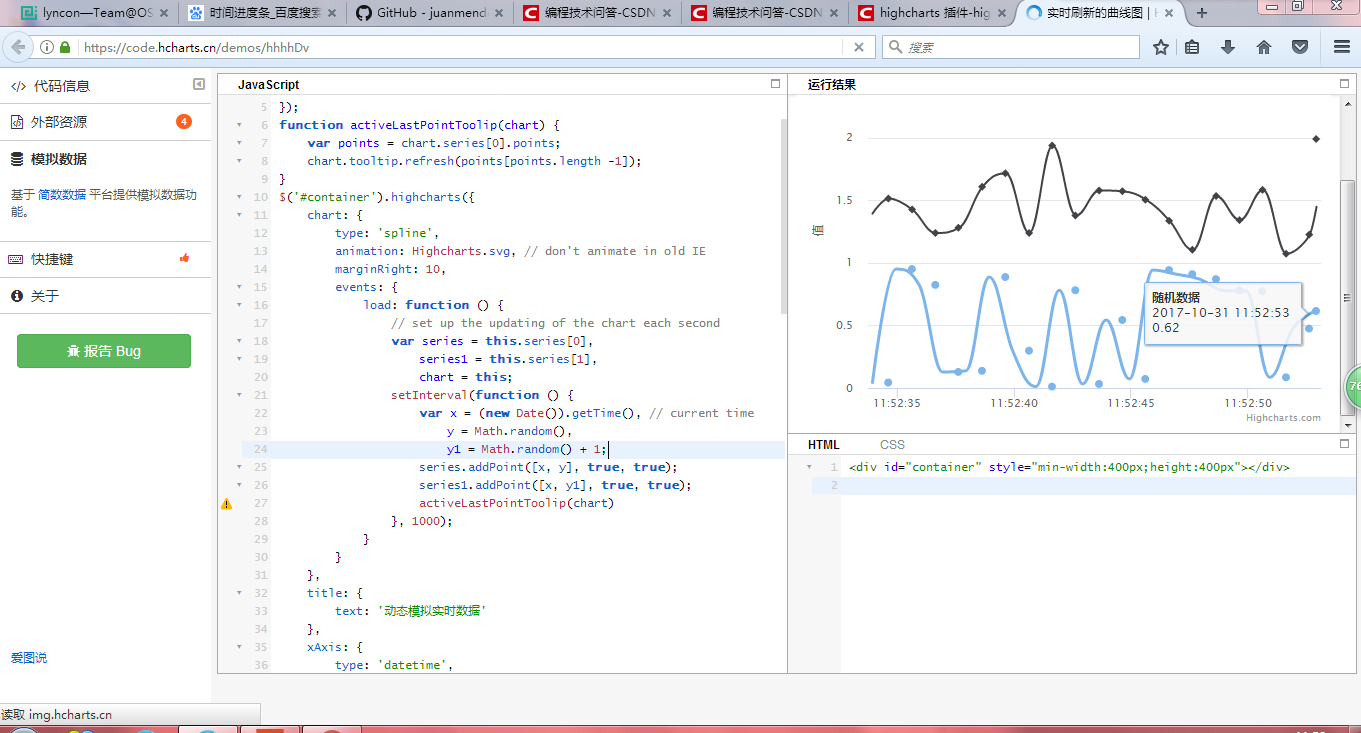2017-10-30 04:28

# highcharts插件在动态曲线图上添加多条曲线时，只有最后一条曲线显示绘制动画

highcharts插件在动态曲线图上添加多条曲线时，只有最后一条曲线显示绘制动画• 写回答
• 好问题 提建议
• 关注问题
• 收藏
• 邀请回答

#### 3条回答默认 最新

•孤芳不自賞 2017-10-30 09:29Highcharts.setOptions({
global: {
useUTC: false
}
});
function activeLastPointToolip(chart) {
var points = chart.series.points;
chart.tooltip.refresh(points[points.length -1]);
}
\$('#container').highcharts({
chart: {
type: 'spline',
animation: Highcharts.svg, // don't animate in old IE
marginRight: 10,
events: {
// set up the updating of the chart each second
var series = this.series,
series2 = this.series,
chart = this;
setInterval(function () {
var x = (new Date()).getTime(), // current time
y = Math.random();
activeLastPointToolip(chart)
}, 1000);
}
}
},
title: {
text: '动态模拟实时数据'
},
xAxis: {
type: 'datetime',
tickPixelInterval: 150
},
yAxis: {
title: {
text: '值'
},
plotLines: [{
value: 0,
width: 1,
color: '#808080'
}]
},
tooltip: {
formatter: function () {
return '' + this.series.name + '
' +
Highcharts.dateFormat('%Y-%m-%d %H:%M:%S', this.x) + '
' +
Highcharts.numberFormat(this.y, 2);
}
},
legend: {
enabled: false
},
exporting: {
enabled: false
},
series: [{
name: '随机数据',
data: (function () {
// generate an array of random data
var data = [],
time = (new Date()).getTime(),
i;
for (i = -19; i <= 0; i += 1) {
data.push({
x: time + i * 1000,
y: Math.random()
});
}
return data;
}())
},
{
name: '随机数据2',
data: (function () {
// generate an array of random data
var data = [],
time = (new Date()).getTime(),
i;
for (i = -19; i <= 0; i += 1) {
data.push({
x: time + i * 1000,
y: Math.random()
});
}
return data;
}())
}]
}, function(c) {
activeLastPointToolip(c)
});

评论
解决 无用
打赏 举报
•毕竟我是萌萌哒啊_ 2017-10-31 03:58两条线可能清楚点。我不知道这是哪里写的有问题。

评论
解决 无用
打赏 举报
•yuncheol_kim 2020-09-19 16:05